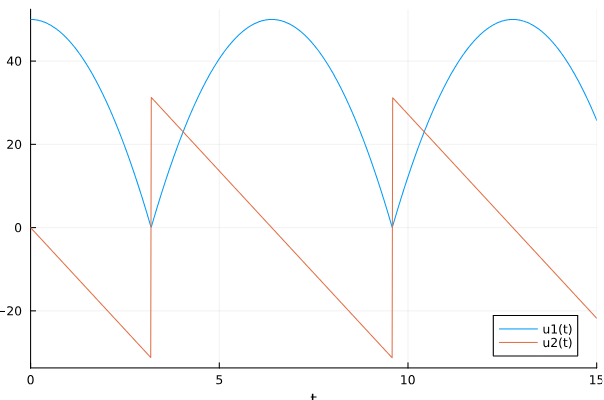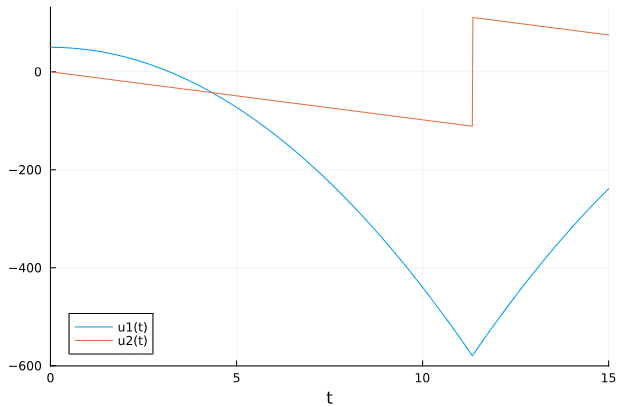# DiscreteCallback does not trigger as expected

I am testing whether DiscreteCallback could be used instead of ContinuousCallback. For testing purposes I modified the ContinuousCallback bouncing ball example from the documentation: Bouncing ball ContinuousCallback example
As an alternative to the ContinuousCallback u==0 I tried to change the condition to trigger when the ball position goes below 0. However, the ball passes below zero with the DiscreteCallback as it does not trigger when the condition u<=0.0 is fulfilled. What is going wrong here?

ContinuousCallback solution (correct):DiscreteCallback solution going below zero:MWE:

``````function f(du, u, p, t)
du = u
du = -p
end

function condition(u, t, integrator) # Event when condition(u,t,integrator) == 0
u
end

function condition_d(u, t, integrator) # Event when condition(u,t,integrator) <= 0
u<=0.0
end

function affect!(integrator)
integrator.u = -integrator.u
end

using DifferentialEquations
cb_c = ContinuousCallback(condition, affect!)
cb_d = DiscreteCallback(condition_d, affect!)

u0 = [50.0, 0.0]
tspan = (0.0, 15.0)
p = 9.8
prob = ODEProblem(f, u0, tspan, p)

sol_c = solve(prob, Tsit5(), callback = cb_c)
sol_d = solve(prob, Tsit5(), callback = cb_d)

using Plots;
plot(sol_d)
plot(sol_c)
``````

It did trigger on the first step end where `u <= 0.0`, just as you asked for. DiscreteCallbacks trigger on the end of steps.

Thanks for your reply, but I am not sure I fully understand why it triggers. What determines the “step end”?

The step end is determined by the `dt` of the stepper.

1 Like
• The `ContinuousCallback` is applied when a given continuous condition function hits zero. This hitting can happen even within an integration step, and the solver must be able to detect it and adjust the integration step accordingly. This type of callback implements what is known in other problem-solving environments as an Event.
• The `DiscreteCallback` is applied when its condition function is `true`, but the condition is only evaluated at the end of every integration step.

So for example, if you are using a fixed time step method and `dt=0.1`, the condition for both are checked at `0.1`, `0,2`, etc. For a ContinuousCallback, its event is defined by a rootfinding condition so it will find the `t` where it “exactly” (up to floating point error) crosses zero. So if `u == 0` at `t = 0.23`, it will step to 0.3 and then pull back to `t = 0.23` to apply the event there. The DiscreteCallback is purely at the step behavior, so it will check at `0.3` and apply the `affect!` at 0.3.

But that means `DiscreteCallback` isn’t able to do things like bouncing ball? Yes, it’s a different tool for a different job. For example, let’s say you wanted to project the end of every step onto some manifold (Manifold Projection · DiffEqCallbacks.jl). “When to project” is “at the end of steps”, so the condition is just `true` and the `affect!` is the projection with a DiscreteCallback. There is no way to define this with a ContinuousCallback, you’d have to do something like “when the solution is x away from the manifold”, which is a different condition.

DiscreteCallback is for things like adding new progress bars to the solve, changing the stepping behavior, applying automated mesh refinement, and stuff like that which is not defined at an exact time but is instead a modification to the solve (or logging behavior). ContinuousCallback is for traditional “event handling”, which is defined by rootfinding functions.

If you use the wrong one for the wrong thing they will not give the behavior you need, and they have different behaviors because they serve two distinctly different purposes.

Hopefully that clarifies.

2 Likes

Thanks, that makes sense and explains why DiscreteCallback is not the right thing to use for my use case.

Coming back to my original problem. I am interested in solving an ODE which only evolves when two ‘larger than’ conditions are fulfilled (in pseudo code):

``````function ODE(du, u, p, t)
...
if f1(u,p)>=0.0 && f2()>=0.0
du = g1(u,p)
du = g2(u,p)
else
du = 0.0
du = 0.0
end
``````

I understand this should be implemented using a `ContinuousCallback(condition, affect!)` as the condition could change from true to false (and vice versa) within a step. Should I formulate my ‘larger-than’ `condition` function for ContinuousCallback using something like `1.0-heaviside(f1)*heaviside(f2)` (evaluates to `0.0` when `f1>=0.0 && f2>=0.0`), and in my `affect!` function set a boolean which is checked in the ODE? And similarly make a `condition` function for ‘smaller-than’ to let `affect!` change said boolean to false? The two conditions should be combined in a `CallbackSet`.
(Unfortunately, I don’t have an MWE that I can share)
Or is the heaviside problematic in the root finding of `ContinuousCallback`? If that’s the case I could instead let `condition` functions check any zero-crossing of `f1` and `f2` and then have an `affect!` function set the boolean upon which the ODE evolves accordingly.

Use a `VectorContinuousCallback` to impose both of those at the same time.